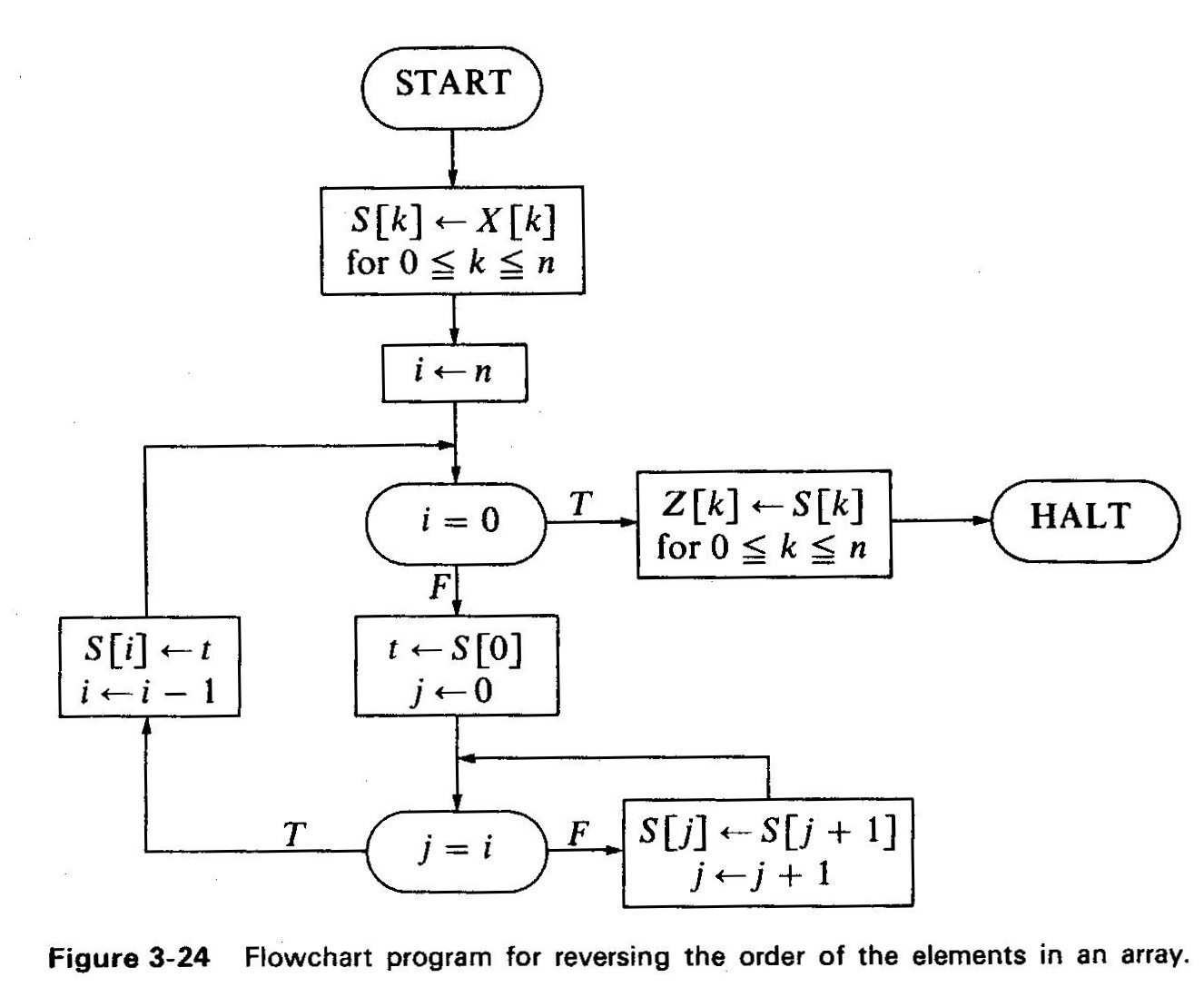# Assignment 2

## 1. Independence on the Cut-Set

Consider program II ("is prime") in assignment 1. After transforming the flowchart to a transition graph we get 4 nodes, and we need 3 cut-points to prove partial correctness.

• Add a new cut-point and formulate its assertion using backwards propagation. Prove partial correctness using the full cut-set.
• Same as above using forward propagation.
• Remove a cut-point (other than the one added) from the full cut-set, and prove partial correctness.

Your claims can depend on your proven claims from assignment 1 (part II) - you don't have to copy them. Example: "seeing that verification condition VC23 holds (proved in assignment 1), we get...". Make sure that your references are clear and readable. When in doubt - copy.

## 2. Verifying Success

Consider program III ("min of array") in assignment 1 after transformed to a transition graph.

• Prove that the resulting program is fault-free.

## 3. Finding Inductive Assertions

The following program takes an array X of n+1, n>=0 elements X, X, ..., X[n] as input, and output an array Z of n+1 elements Z, Z, ..., Z[n] where the elements of Z are the elements of X in reverse order. This means: Z = X[n], Z = X[n-1], ..., Z[n] = X.

Specification:

• Phi(n,X): n >=0
• Psi(n,X,Z): for all k (where 0 =< k =< n), Z[x] = X[n-k].Transform the flowchart into a transition graph. Use the heuristics studied in class to find the invariants for the cut-points. For each invariant specify which heuristics was used. Specify if invariants obtained using the heuristics were strong enough, or you had to strengthen them to be able to prove partial correctness.

## Hand-in and due date

Sunday 30/3/2003 (hand-in in my box #108).